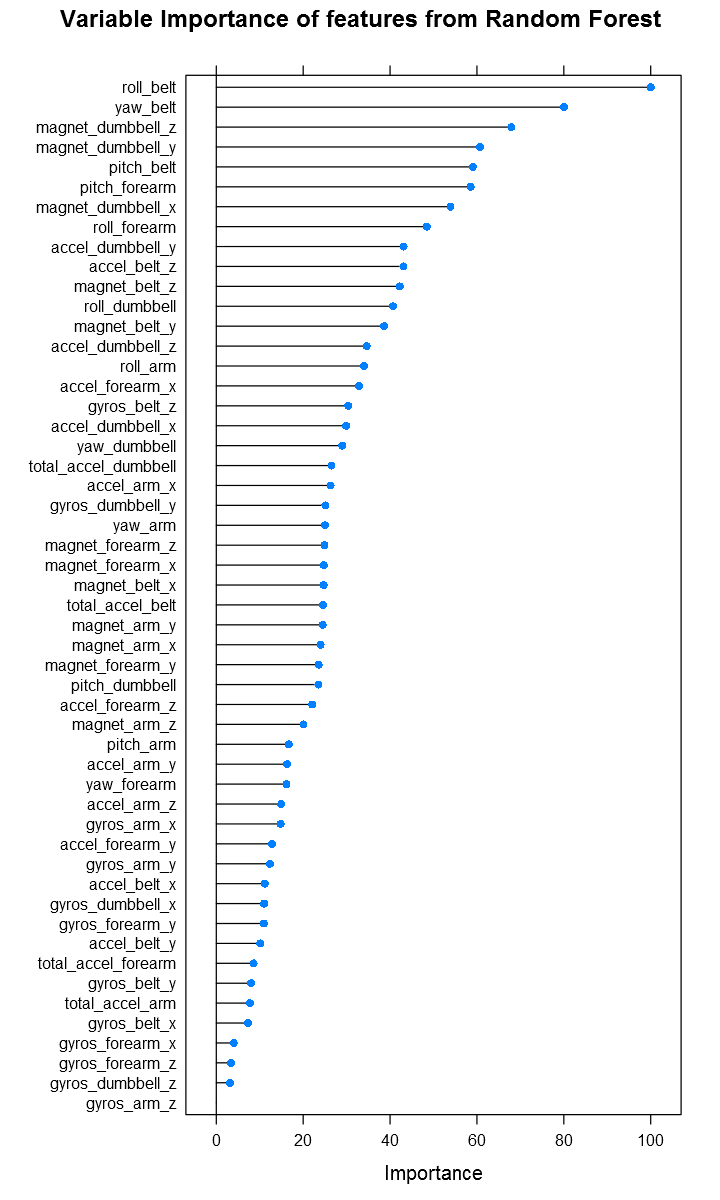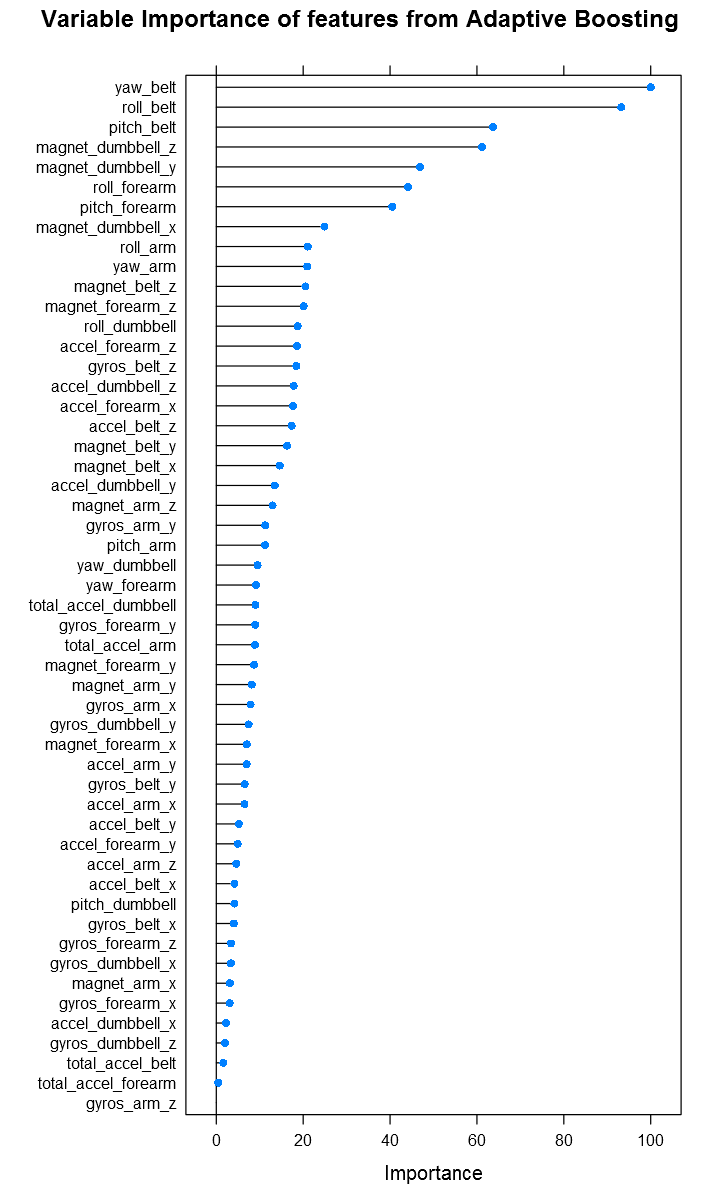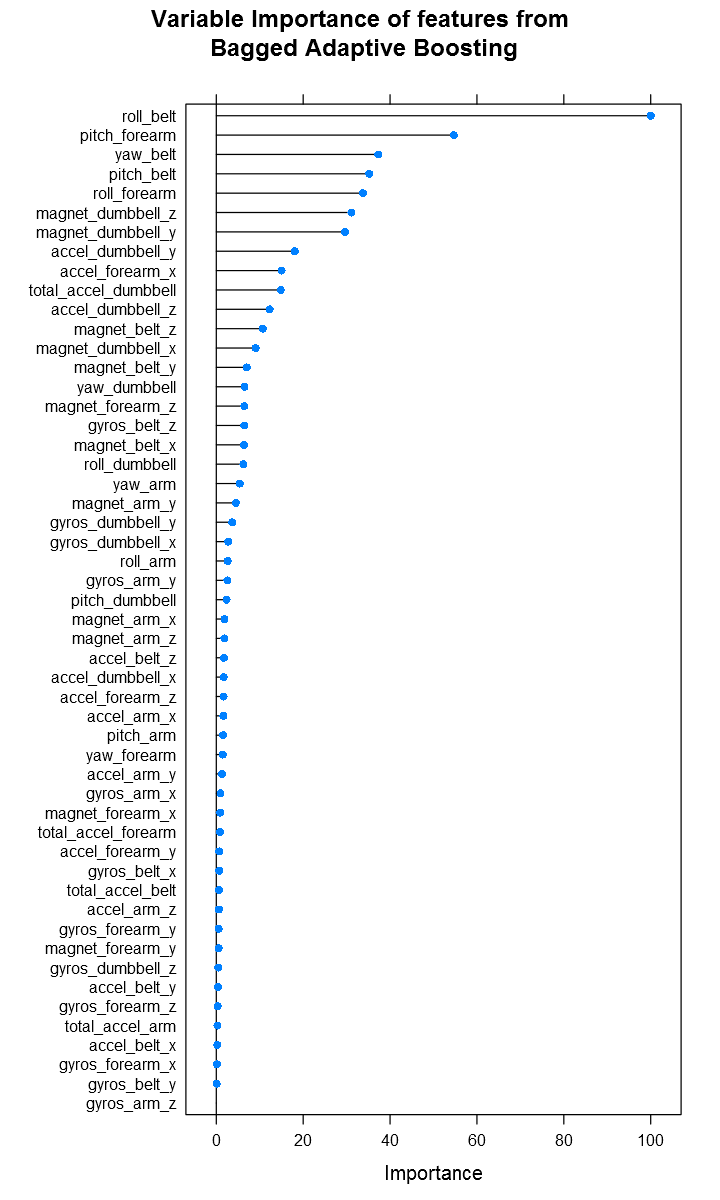# Fisseha Berhane, PhD

#### Data Scientist443-970-2353[email protected] CV Resume### Summary¶

In this project, ensemble tree-based predictive models that determine the manner an exercise is performed are built. The models used are Random Forest, Adaptive Boosting and Bagged Adaptive Boosting. Data from accelerometers on the belt, forearm, arm, and dumbell of 6 participants, who were asked to perform barbell lifts correctly and incorrectly in 5 different ways, are employed to predict whether a particular exercise is performed correctly or not.

### Data retrieval and exploration¶

The dataset used for building the ensemble tree-based models can be downloaded from here. First, let's download the dataset from the provided link and divide it into two subsets: one for training, building and evaluating the models and the second subset to evaluate out of sample error.

According to the rule of thumb for prediction study design, I partitioned the data into 60% training and 40% testing. Then, I put aside the testing data and finished the model building process using the training data. Before building the model, I made exploration on the training data. I also tried to understand the covariates (features) and their associations. The paper by Velloso et al. (2013) explains the covariates. The data for this project come from this source: http://groupware.les.inf.puc-rio.br/har.

In :
# Load required libraries

require(ggplot2)
library(caret)
library(nnet)
library(NeuralNetTools)
library(plotmo)
library(ROCR)
library(dplyr)

In :
data1<-read.csv("http://d396qusza40orc.cloudfront.net/predmachlearn/pml-training.csv",na.strings=c("NA",""))


Now, let's see the dimensions of the dataset and the variables.

In :
dim(data1)

Out:
1. 19622
2. 160

Then, I divided the dataset into training and testing subsets.

In :
for_training<-createDataPartition(y=data1$classe,p=0.6,list=FALSE) training <-data1[for_training,] testing <-data1[-for_training,]  ### Explore the training data¶ In : dim(training) tail(names(training)) # to see some of the features  Out: 1. 11776 2. 160 The training data has 160 columns (variables) and 11776 rows (observations). The predictand (dependent) variable is “classe”, which is the right most column. Then, I removed the covariates which are not important for the machine learning algorithm development. These are the row number(X), user_name, raw_timestamp_part1, raw_timestamp_part2, cvtd_timestamp, new_window and num_window. It is vital to keep in mind that the testing data is not used in any way for model development. The model building is done entirely based on the training data. Remove data not important for model development In : training<-training[,8:160]  Many of the covariates have lots of missing data. Those with missing data are removed. In : non_missing_training<-apply(!is.na(training),2,sum)>=dim(training) training<-training[,non_missing_training] dim(training)  Out: 1. 11776 2. 53 After removing the variables with missing data, I am left with 52 features and the dependent variable (53 columns in total). ### Predictive Model Building¶ Random forest, along with Boosting, is one of the most accurate classifiers. Random Forest is designed to improve prediction accuracy of CART. It works by building a large number of CART trees. To make a prediction for a new observation, each tree “votes” on the outcome, and we pick the outcome that receives the majority of the votes. More information can be found on Wikipedia. On many problems, the performance of random forests is very similar to boosting (Hastie et al., 2005). Ensemble tree techniques are suitable for this project dataset because: (a) they handle non-linearity (b) they handle large inputs whose interactions is not understood, (c) they handle unscaled inputs and categorical data, (d) they help to visually examine how the predictors are contributing into the final model by extracting the model output trees. Here, we are using Random Forest, Adaptive Boosting and Bagged Adaptive Booting. The caret package is employed to build and evaluate the various models considered. ### Random Forest¶ Let's use 10 fold cross validation In : set.seed(3333) # this is set to ensure the reproducibility of the results rf_fit<-train(classe~.,data=training,method="rf", allowParallel=TRUE,prox=TRUE, trControl=trainControl(method="cv",number=10))  Let's see the importance of the covariates. In : importance_rf <- varImp(rf_fit) plot(importance_rf, main = "Variable Importance of features from Random Forest")  Out:or we can have a look at the top 20 important features: In : importance_rf  Out: rf variable importance only 20 most important variables shown (out of 52) Overall roll_belt 100.00 yaw_belt 80.04 magnet_dumbbell_z 67.92 magnet_dumbbell_y 60.74 pitch_belt 59.04 pitch_forearm 58.54 magnet_dumbbell_x 53.92 roll_forearm 48.47 accel_dumbbell_y 43.11 accel_belt_z 43.08 magnet_belt_z 42.22 roll_dumbbell 40.67 magnet_belt_y 38.64 accel_dumbbell_z 34.62 roll_arm 33.96 accel_forearm_x 32.80 gyros_belt_z 30.38 accel_dumbbell_x 29.89 yaw_dumbbell 29.01 total_accel_dumbbell 26.52 Then let us see the confusion matrix to understand how the model performs: In : options(digits=2) rf_fit$finalModel$confusion  Out: ABCDEclass.error A334520019e-04 B19224812000.014 C0212028500.013 D0041188630.023 E000521600.0023 The confusion matrix shows that the model is doing a good job in fitting the training data set. The summary of the model is below: In : rf_fit  Out: Random Forest 11776 samples 52 predictor 5 classes: 'A', 'B', 'C', 'D', 'E' No pre-processing Resampling: Cross-Validated (10 fold) Summary of sample sizes: 10597, 10598, 10599, 10598, 10598, 10599, ... Resampling results across tuning parameters: mtry Accuracy Kappa Accuracy SD Kappa SD 2 0.9901506 0.9875387 0.004180135 0.005289414 27 0.9896413 0.9868945 0.003894178 0.004927570 52 0.9822516 0.9775446 0.004608570 0.005828354 Accuracy was used to select the optimal model using the largest value. The final value used for the model was mtry = 2.  ### Cross-validation¶ Similar to what was done to the training data, the first eight columns and those with missing covariates are removed from the testing data and the rest covariates are used for prediction. In : testing <-testing[,8:160]  Similar to what was done in the training data, remove covariates with NAs In : non_missing_testing<-apply(!is.na(testing),2,sum)==dim(testing) testing<-testing[,non_missing_testing] dim(testing)  Out: 1. 7846 2. 53 predict using the model built in with the training data In : prediction_rf<-predict(rf_fit,testing)  In : accuracy_rf = sum(prediction_rf==testing$classe)/length(testing$classe) cat("The accuracy from Random Forest Model is ",round(accuracy_rf,3))  The accuracy from Random Forest Model is 0.99 We see that Random Forest has very good accuracy with error of less than 1% ### Predicting with Random Forest Model¶ Now, let's download this dataset and make predictions using the model built above. Remove the first seven columns which are not covariates for the model and also remove covariates with missing values In : test_given<-read.csv("http://d396qusza40orc.cloudfront.net/predmachlearn/pml-testing.csv",na.strings=c("NA","")) test_given<-test_given[,8:160] non_missing_test<-apply(!is.na(test_given),2,sum)>=dim(test_given) test_given<-test_given[,non_missing_test]  Predict for the test data and save the result In : prediction = predict(rf_fit,test_given)  ##### Saving prediction results¶ In : pml_write_files = function(x){ n = length(x) for(i in 1:n){ filename = paste0("problem_id_",i,".txt") write.table(x[i],file=filename,quote=FALSE,row.names=FALSE,col.names=FALSE) } } pml_write_files(prediction)  The model predicts the test data 100% accurate. ### Adaptive Boosting¶ In : cctrl <- trainControl(method = "cv", number = 10, returnResamp = "all", classProbs = TRUE) grid <- expand.grid(mfinal = (1:20), maxdepth = c(1:10), coeflearn = c("Breiman", "Freund", "Zhu")) set.seed(100) my_adaboost <- train(classe~., data=training, method = "AdaBoost.M1", trControl = cctrl, tuneGrid = grid, metric = "Accuracy")  In : importance_adaboost <- varImp(my_adaboost) plot(importance_adaboost, main = "Variable Importance of features from Adaptive Boosting")  Out:In [ ]: importance_adaboost  In : my_adaboost$bestTune

Out:
mfinalmaxdepthcoeflearn
4002010Freund
In :
prediction_adaboost<-predict(my_adaboost,testing)

In :
accuracy_adaboost = sum(prediction_adaboost==testing$classe)/length(testing$classe)

The accuracy from Adaptive Boosting Model is  0.992

We see that Adaptive Boosting has similar accuracy with Random Forest.

In :
cctrl <- trainControl(method = "cv", number = 10, returnResamp = "all",
classProbs = TRUE)

grid <- expand.grid(mfinal = (1:20), maxdepth = c(1:10))

set.seed(100)

trControl = cctrl,
tuneGrid = grid,
metric = "Accuracy",
preProc = c("center", "scale"))

In :
importance_adabag <- varImp(my_adabag)

Out:

Out:
AdaBag variable importance

only 20 most important variables shown (out of 52)

Overall
roll_belt            100.000
pitch_forearm         54.672
yaw_belt              37.292
pitch_belt            35.201
roll_forearm          33.735
magnet_dumbbell_z     31.104
magnet_dumbbell_y     29.625
accel_dumbbell_y      18.075
accel_forearm_x       15.026
total_accel_dumbbell  14.830
accel_dumbbell_z      12.268
magnet_belt_z         10.675
magnet_dumbbell_x      9.058
magnet_belt_y          7.005
yaw_dumbbell           6.494
magnet_forearm_z       6.461
gyros_belt_z           6.437
magnet_belt_x          6.371
roll_dumbbell          6.246
yaw_arm                5.416In :
my_adabag$bestTune  Out: mfinalmaxdepth 1971710 In : prediction_adabag<-predict(my_adabag,testing) accuracy_adabag = sum(prediction_adabag==testing$classe)/length(testing\$classe)


The accuracy from Bagged Adaptive Boosting Model is  0.882

## Conclusion¶

In this project, we saw the performance of various ensemble tree-based machine learning techniques in recognizing human activity. We considered Random Forest, Adaptive Boosting and Bagged Adaptive Boosting. We used 10-fold cross-validation to evaluate the performance of the models. All models have very high accuracy with errors of about 1%.

Hastie, T., R. Tibshirani, J. Friedman, and J. Franklin, 2005: The elements of statistical learning: data mining, inference and prediction. The Mathematical Intelligencer, 27, 83-85.

Velloso, E., A. Bulling, H. Gellersen, W. Ugulino, and H. Fuks, 2013: Qualitative activity recognition of weight lifting exercises. Proceedings of the 4th Augmented Human International Conference, ACM, 116-123.